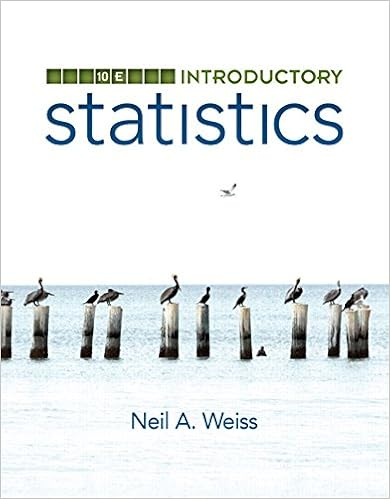# Exercise 914 let f x y sin x y determine the

• Parrish101
• 316
• 80% (5) 4 out of 5 people found this document helpful

This preview shows page 274 - 277 out of 316 pages.

Exercise 9.1.4.Let f(x,y)=sin(xy). Determine the equations and shapes of the cross-sections whenx=0, y=0, x=y, and describe the level curves. Use a three-dimensional graphing tool to graph thesurface.Exercise 9.1.5.Let f(x,y)=(x2y2)2. Determine the equations and shapes of the cross-sections whenx=0, y=0, x=y, and describe the level curves. Use a three-dimensional graphing tool to graph thesurface.Exercise 9.1.6.Find the domain of each of the following functions of two variables:(a)radicalbig9x2+radicalbigy24(b)arcsin(x2+y22)(c)radicalbig16x24y2Exercise 9.1.7.Below are two sets of level curves. One is for a cone, one is for a paraboloid. Which iswhich? Explain.9.2 Limits and ContinuityTo develop calculus for functions of one variable, we needed to make sense of the concept of a limit,which was used in the definition of a continuous function and the derivative of a function. Limits involvingfunctions of two variables can be considerably more difficult to deal with; fortunately, most of the functionswe encounter are fairly easy to understand.The potential difficulty is largely due to the fact that there are many ways to “approach” a point in thex-yplane. If we want to say thatlim(x,y)(a,b)f(x,y)=L, we need to capture the idea that as(x,y)gets closeto(a,b)thenf(x,y)gets close toL. For functions of one variable,f(x), there are only two ways thatxcan
##### We have textbook solutions for you!The document you are viewing contains questions related to this textbook.
Chapter 9 / Exercise 89
Mathematical Excursions
AufmannExpert VerifiedBrowse all Textbook Solutions
9.2. Limits and Continuity267approacha: from the left or right. But there are an infinite number of ways to approach(a,b): along anyone of an infinite number of straight lines, or even along a curved path in thex,y-plane. We might hopethat it’s really not so bad—suppose, for example, that along every possible line through(a,b)the value off(x,y)gets close toL; surely this means that “f(x,y)approachesLas(x,y)approaches(a,b)”. Sadly, no.Figure 9.3:f(x,y)=xy2x2+y4Example 9.6: Weird LimitAnalyzef(x,y)=xy2/(x2+y4).Solution.Whenx=0 ory=0,f(x,y)is 0, so the limit off(x,y)approaching the origin along eitherthexoryaxis is 0. Moreover, along the liney=mx,f(x,y)=m2x3/(x2+m4x4). Asxapproaches 0this expression approaches 0 as well. So along every line through the originf(x,y)approaches 0. Nowsuppose we approach the origin alongx=y2. Thenf(x,y)=y2y2y4+y4=y42y4=12,so the limit is 1/2. Looking at Figure9.3, it is apparent that there is a ridge abovex=y2. Approaching
268Partial Differentiationthe origin along a straight line, we go over the ridge and then drop down toward 0, but approaching alongthe ridge the height is a constant 1/2.Fortunately, we can define the concept of limit without needing to specify how a particular point isapproached—indeed, in Definition3.3, we didn’t need the concept of “approach.” Roughly, that definitionsays that whenxis close toathenf(x)is close toL; there is no mention of “how” we get close toa. Wecan adapt that definition to two variables quite easily:Definition 9.1: Limit of a Multivariate FunctionSupposef(x,y)

Upload your study docs or become a

Course Hero member to access this document

Upload your study docs or become a

Course Hero member to access this document

End of preview. Want to read all 316 pages?

Upload your study docs or become a

Course Hero member to access this document

Term
Fall
Professor
N/A
##### We have textbook solutions for you!
The document you are viewing contains questions related to this textbook.The document you are viewing contains questions related to this textbook.
Chapter 9 / Exercise 89
Mathematical Excursions
AufmannExpert Verified
•••